# 5. (a) Show that Q(V2) C Q V2). (b) Find [Q( 12): Q(V2)]. (c) Show that...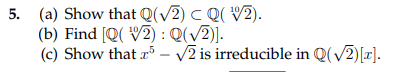5. (a) Show that Q(V2) C Q V2). (b) Find [Q( 12): Q(V2)]. (c) Show that r - V2 is irreducible in Q(V2)[].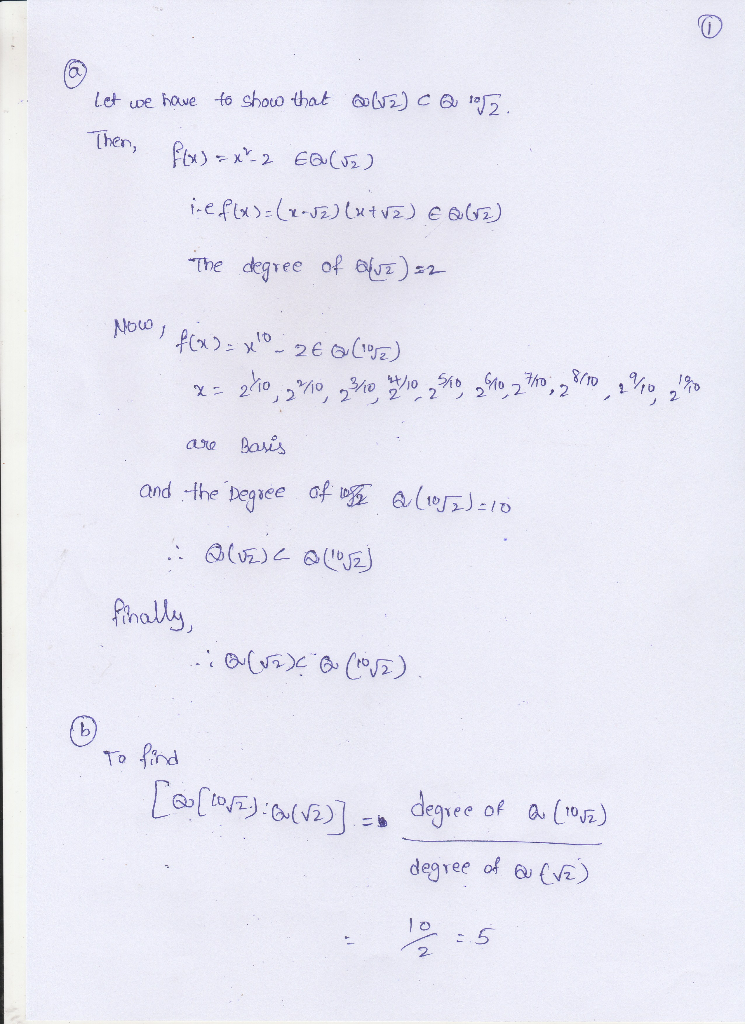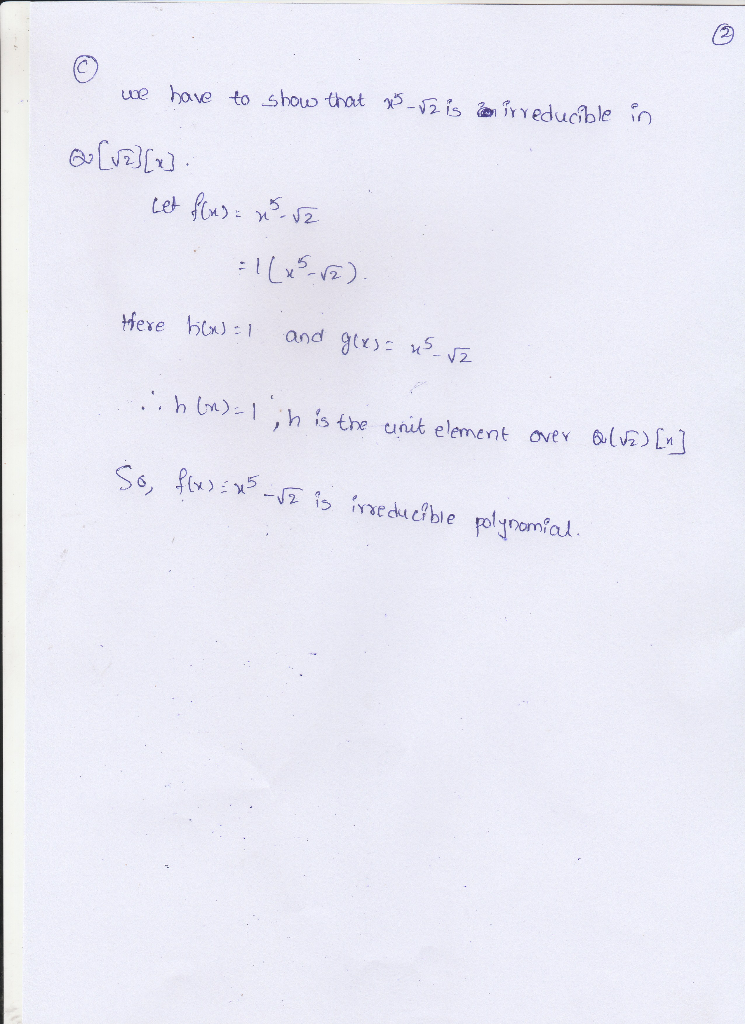#### Earn Coin

Coins can be redeemed for fabulous gifts.

Similar Homework Help Questions
• ### 2.(b) Design a constructible number of the form r for some r E Q(V2) 2.(c) Is V2 in a tower of fi...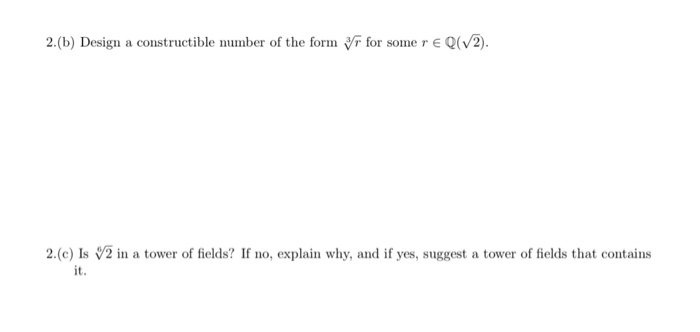2.(b) Design a constructible number of the form r for some r E Q(V2) 2.(c) Is V2 in a tower of fields? If no, explain why, and if yes, suggest a tower of fields that contains it. 2.(b) Design a constructible number of the form r for some r E Q(V2) 2.(c) Is V2 in a tower of fields? If no, explain why, and if yes, suggest a tower of fields that contains it.

• ### Show that the following polynomials are irreducible over Q. (a) (8 points) f(1) = 5.rº –...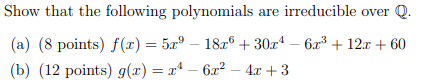Show that the following polynomials are irreducible over Q. (a) (8 points) f(1) = 5.rº – 1826 + 30x4 – 6r2 + 12x + 60 (b) (12 points) g(x) = r" - 6.12 – 4.: +3

• ### 3. (25 points) Show that if A (R,,0,1), then A must contain Q and v2.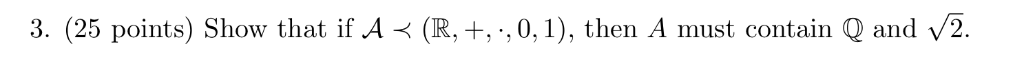3. (25 points) Show that if A (R,,0,1), then A must contain Q and v2. 3. (25 points) Show that if A (R,,0,1), then A must contain Q and v2.

• ### 10. Let p(x) +1. Let E be the splitting field for p(x) over Q. a. Find the resolvent cubic R(z). ...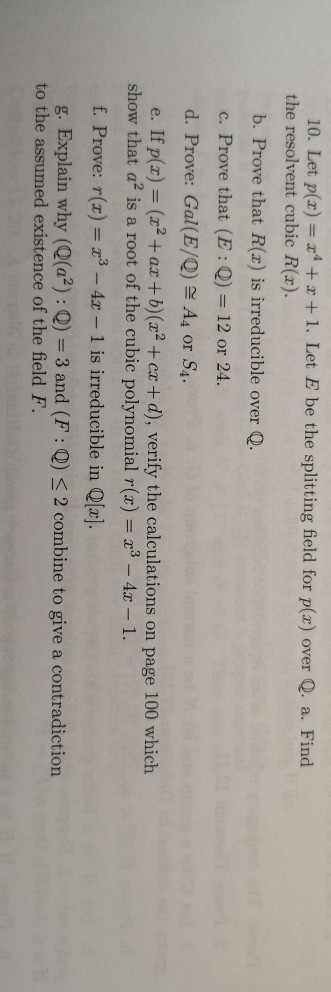Part D,E,F,G 10. Let p(x) +1. Let E be the splitting field for p(x) over Q. a. Find the resolvent cubic R(z). b. Prove that R(x) is irreducible over Q. c. Prove that (E:Q) 12 or 24. d. Prove: Gal(E/Q) A4 or S4 e. If p(x) (2+ az+ b)(a2 + cr + d), verify the calculations on page 100 which show that a2 is a root of the cubic polynomial r(x)3-4. 1. f. Prove: r(x) -4z 1 is irreducible in...

• ### Show that the irreducible polynomial x4 - 2 over Q, has roots a, b, c in its splitting field such...

Show that the irreducible polynomial x4 - 2 over Q, has roots a, b, c in its splitting field such that the fields Q(a, b) and Q(a, c) are not isomorphic over Q (Hint: The roots are (4√2, -4√2, 4√2i, -4√2i), and the splitting field is Q(4√2, i,).)

• ### Let R={1 € Q : [0) € Z}. (a) Show that R is an integral domain...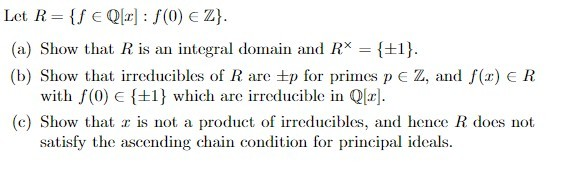Let R={1 € Q : [0) € Z}. (a) Show that R is an integral domain and R* = {+1}. (b) Show that irreducibles of Rare Ep for primes pe Z, and S() ER with (0 €{+1} which are irreducible in Q[r]. (c) Show that r is not a product of irreducibles, and hence R does not satisfy the ascending chain condition for principal ideals.

• ### (1) Prove that QV2+3) Q(V2, V3) (2) Prove that (Q(V2, v3):Q) 4 (3) Find the minimal...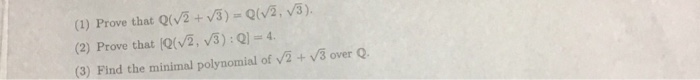(1) Prove that QV2+3) Q(V2, V3) (2) Prove that (Q(V2, v3):Q) 4 (3) Find the minimal polynomial of V2 + V/3 overQ.

• ### 5. Let V2 be the real cube root of two. Set e: -1+Bi (i) Show that 2, V2e, and 2e2 are the distin...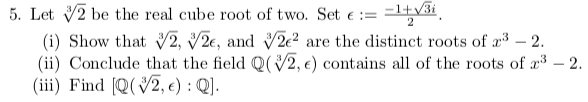all of (i) (ii) (iii) 5. Let V2 be the real cube root of two. Set e: -1+Bi (i) Show that 2, V2e, and 2e2 are the distinct roots of 32 (ii) Conclude that the field Q(2,) contains all of the roots of 3 -2. (ii) Find (Q(V2,e):Q] 5. Let V2 be the real cube root of two. Set e: -1+Bi (i) Show that 2, V2e, and 2e2 are the distinct roots of 32 (ii) Conclude that the field Q(2,)...

• ### B. Let p and q be distinct positive prime numbers. Set a p+ (a) Find a...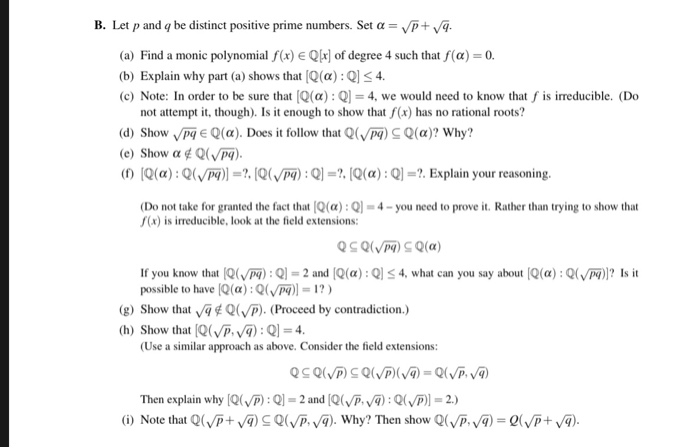B. Let p and q be distinct positive prime numbers. Set a p+ (a) Find a monic polynomial f(x) EQlr of degree 4 such that f(a) 0. (b) Explain why part (a) shows that (Q(a):QS4 (c) Note: In order to be sure that IQ(α) : Q-4, we would need to know that f is irreducible. (Do not attempt it, though). Is it enough to show that f(x) has no rational roots? (d) Show V pg E Q(α). Does it follow...

• ### Exercise 3. Let u2= (5) C) V2 = V1 = and E u1, u2},F = {v1,v2}...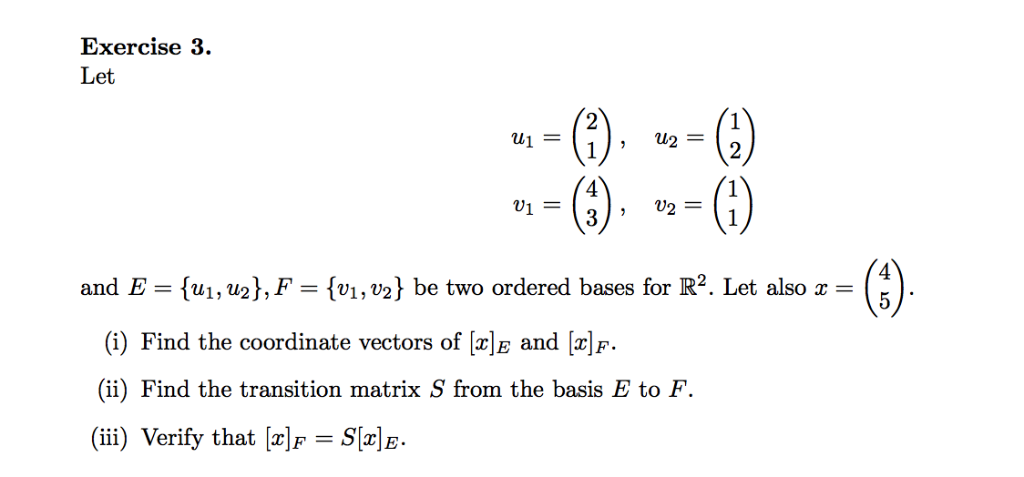Exercise 3. Let u2= (5) C) V2 = V1 = and E u1, u2},F = {v1,v2} be two ordered bases for R2. Let also 5 (i) Find the coordinate vectors of [x]E and [x\f. (ii) Find the transition matrix S from the basis E to F. (ii) Verify that [x]f = S[r]E Exercise 3. Let u2= (5) C) V2 = V1 = and E u1, u2},F = {v1,v2} be two ordered bases for R2. Let also 5 (i) Find the...

Free Homework App